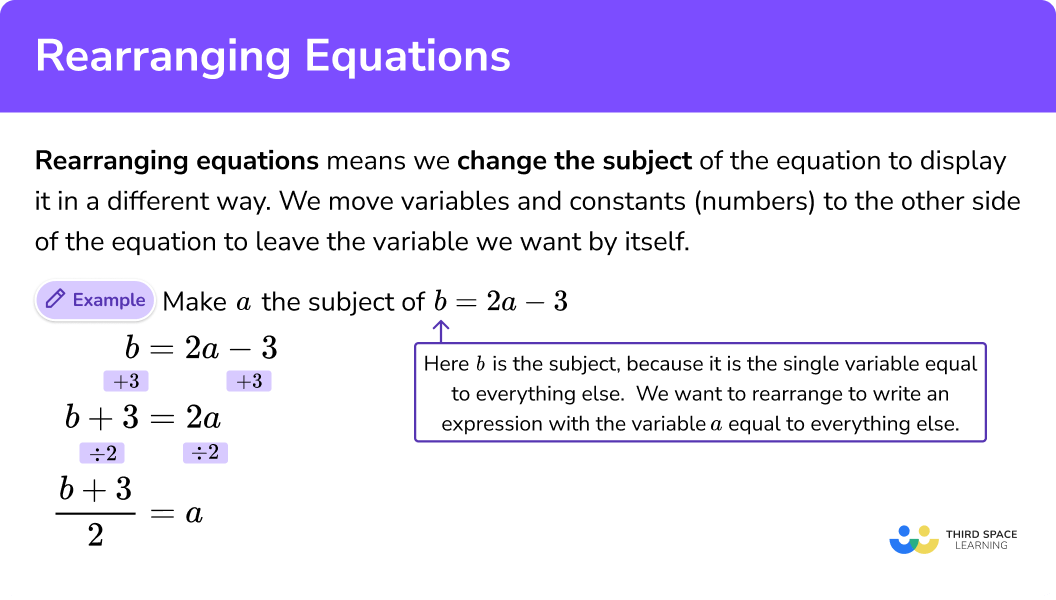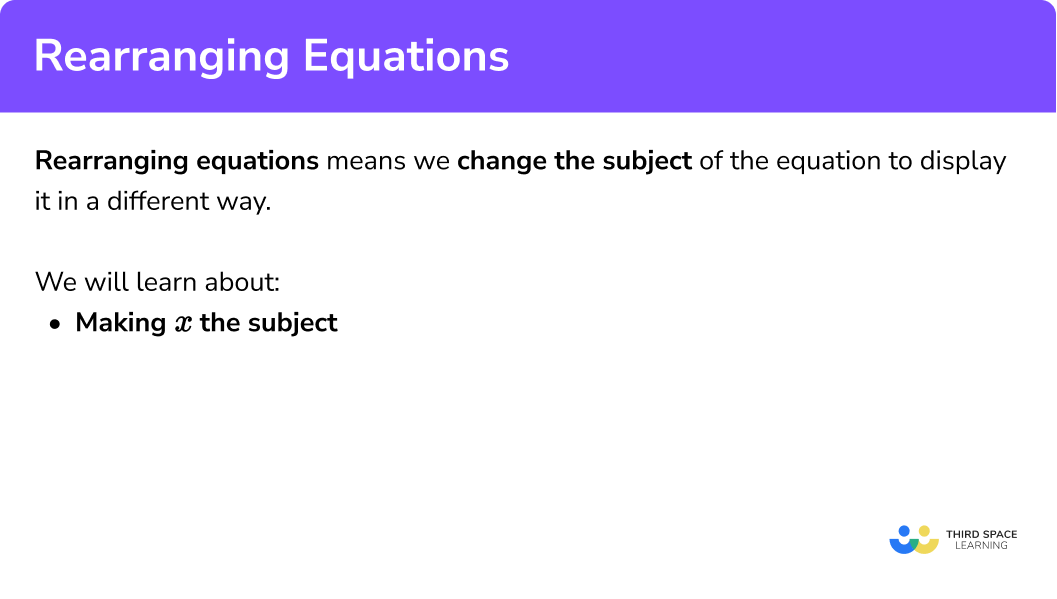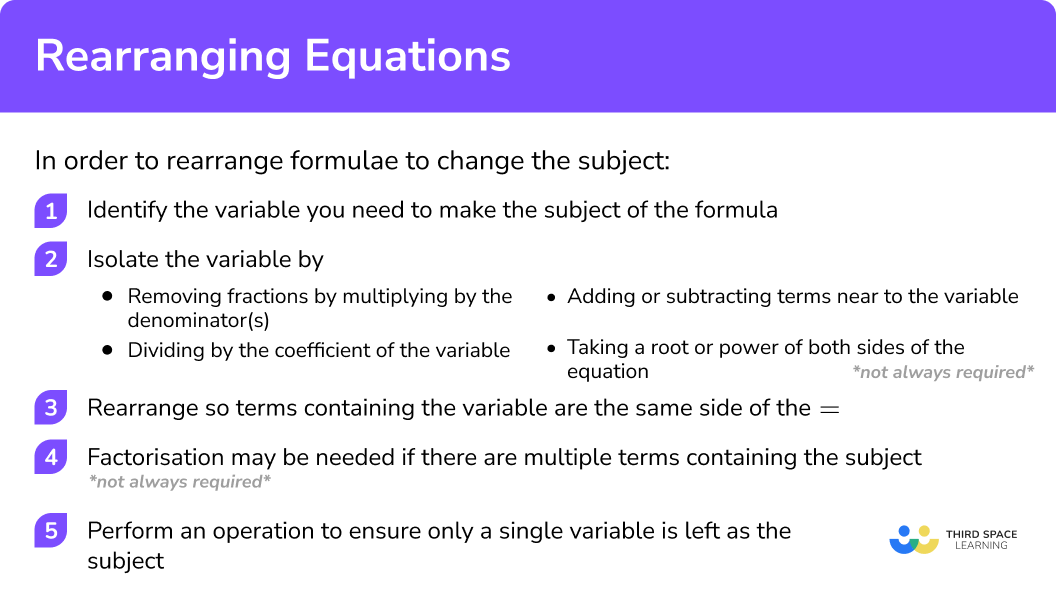GCSE Maths Algebra Equations

Rearranging Equations

# Rearranging Equations

Here is everything you need to know about rearranging equations for GCSE maths (Edexcel, AQA and OCR). You’ll learn what rearranging equations means and how to change the subject of the formula.

Look out for the rearranging equations worksheets and exam questions at the end.

## What is rearranging equations?

Rearranging equations changes the form of the equation to display it in a different way. This is sometimes called changing the subject.

How to rearrange equations step by step:

1. Identify the variable you need to make the subject.
2. Isolate the variable by: removing any fractions by multiplying by the denominator/s, dividing by the coefficient of the variable, and by square rooting or squaring both sides of the equation
3. Perform inverse operations so that each term that needs to be made the subject is on one side of the equation.

## What does rearranging equations mean?

When we rearrange an equation we change the form of the equation to display it in a different way.

For example, the below three equations are rearranged forms of exactly the same equation.

\begin{aligned} a-b &=2 \\ a &=b+2 \\ a-2 &=b \end{aligned}

Typically we rearrange equations and formulas by using inverse operations to make one variable the subject of the formula. The subject of the formula is the single variable that is equal to everything else. i.e. the term by itself on one side of the equal sign.

Here are some example where s is the subject of the formula.

$s=4+a \qquad\qquad s=5 f-6 c+8 \qquad s=\frac{5+t}{y-8}$

To do this we move variables and constants (numbers) to the other side of the equation from the variable we are trying to make the subject of the formula.

Step-by-step guide: Make x the subject

### What do we mean by rearranging equations?### What do we mean by rearranging equations?## How to rearrange formula to change the subject of the formula

In order to do rearrange formula to change the subject of the formula, I need to follow the steps:

1. Identify the variable you need to make the subject of the formula.
2. Isolate the variable – this step may look slightly different depending on the format of the question.
– Remove any fractions by multiplying by the denominator/s
– Divide by the coefficient of the variable
– Square root or square both sides of the equation
*not always required*
3. Rearrange the equation so each term containing the term you want to be the subject is on one side of the equation – normally the left-hand side.
4. Factorisation may be needed if you have multiple different terms containing your subject e.g. factorise 2x + 3xy to x(2 + 3y)
*not always required*
5. Perform an operation to ensure only the single variable is left as the subject.

### How to rearrange formula to change the subject of the formula## Rearranging equations examples

### Example 1: multiple step but with single variable

p = 2(x − 3)

1. Identify the variable to be made the subject.

$p = 2(x − 3)$

In this question it is x.

2 Divide each side of the equation by 2

3 Add 3 to each side of the equation

$\frac{p}{2}+3= x$

### Example 2: questions involving x2

$y=x^{2}-4$

Identify the variable to be made the subject.

Add 4 to each side of the equation.

Square root each side. Remember: the square root can be a + or -.

### Example 3: questions involving √x

$y=\sqrt{3 x}+n$

Identify the variable to be made the subject.

Subtract ‘n’ from each side of the equation.

The inverse operation of ‘square root’ is to ‘square’ each side.

Divide each side by the equation by the ‘coefficient of x‘. Here the coefficient is 3. Note in this question step 4 was not required.

### Example 4: factorisation of the variable is required

$y=\frac{2 x z}{x-5}$

Identify the variable to be made the subject.

Multiply each side of the equation by the denominator.

Expand the bracket on the left hand side of the equation. This will help us get all terms with x onto one side of the equation.

If we factorise the left side of the equation we will be left with only one of the variable x.

Divide by (y - 2z). This will leave x as the subject of the equation.

### Example 5: factorisation of the variable is required

$\frac{a}{3}=\frac{2-7 x}{x-5}$

Identify the variable to be made the subject.

As in the previous example, we are multiplying the equation by the denominator. In this example we have denominators on both sides we multiply by both.

Expand the bracket on the LHS and RHS of the equation. This will help to get all terms with x onto one side of the equation.

If we factorise the left side of the equation we will be left with only one of the variable x

Now divide by (a+21). This will leave x as the subject of the equation.

### Common misconceptions

• When we perform an operation to one side of the equation we have to do to the other.
• Incorrect use of the inverse operation.
• Incorrectly following the order of operations.
• All variables of the subject need to be on one side of the equal sign.
$E.g. x = 2x+2$

• When we square root a number/variable the answer can be positive or negative.
$E.g. \sqrt{4}=\pm{2}$
√x should be written as ± √x

• Not factorising when we have the subject in more than one term.
E.g. Make x the subject
\begin{aligned} 2x+3xy&=3y\\ x(2+3y)&=3y\\ x&=\frac{3y}{2+3y} \end{aligned}

### Practice rearranging equations questions

1. Make a the subject of the formula h=3(a+7)

h -7=a\frac{h}{3} -7=a\frac{h}{3} -21=ah-21=ah=3(a+7)

Divide both sides by 3

\frac{h}{3}=a+7

Subtract 7 from both sides

\frac{h}{3}-7=a

2. Make b the subject of the formula p=b^{2}-9 k

p+9k=b\pm \sqrt{p+9 k}=b\pm \sqrt{p-9 k}=b\pm \sqrt{9k-p}=bp=b^{2}-9k

p+9k=b^{2}

Square root both sides

\pm \sqrt{p+9 k}=b

3. Make c the subject of the formula g=\sqrt{5 c-r}

g^{2}+r=c\frac{g^{2}-r}{5}=c\frac{g^{2}+r}{5}=cg^{2}-r=cg=\sqrt{5 c-r}

Square both sides

g^{2}=5c-r

g^{2}+r=5c

Divide both sides by 5

\frac{g^{2}+r}{5}=c

4. Make d the subject of the formula y=\frac{3d+1}{4d}

d=\frac{1}{4y}-3d=\frac{1}{4y-3}d=\frac{1}{4y+3}d=\frac{1}{4y}+3y=\frac{3d+1}{4d}

Multiply both sides by 4d

4dy=3d+1

Subtract 3d from both sides

4dy-3d=1

Factorise the left hand side

d(4y-3)=1

Divide both sides by 4y-3

d=\frac{1}{4y-3}

5. Make e the subject of the formula \frac{q}{3} = \frac{6-2e}{e+1}

e=\frac{18-q}{q-6}e=\frac{18-q}{q+6}e=\frac{18+q}{q+6}e=\frac{18-q}{6}\frac{q}{3} = \frac{6-2e}{e+1}

Multiply both sides by 3(e+1)

qe+q=18-6e

Subtract q , and add 6e to both sides

qe+6e=18-q

Factorise the left hand side

e(q+6)=18-q

Divide both sides by q+6

e=\frac{18-q}{q+6}

6. Make f the subject of the formula \frac{l^{3}}{5} = \frac{4l-3f}{2f+9}

f=\frac{20 l+9 l^{3}}{2 l^{3}+15}f=\frac{20 l-9 l^{3}}{2 l^{3}+15}f=\frac{20 l-9 l^{3}}{2 l^{3}-15}f=\frac{20 l+9 l^{3}}{2 l^{3}-15}\frac{l^{3}}{5} = \frac{4l-3f}{2f+9}

Multiply both sides by 5(2f+9)

2fl^{3}+9l^{3}=20l-15f

Add 15f , and subtract 9l^3 , from both sides

2fl^{3}+15f=20l-9l^{3}

Factorise the left hand side

f(2l^{3}+15)=20l-9l^{3}

Divide both sides by 2l^3+15

f=\frac{20 l-9 l^{3}}{2 l^{3}+15}

### Rearranging equations GCSE questions

1. Make x the subject of the formula

$y=2x+4$

$y-4=2x$

(− 4) to make 2x the subject (1)

$\frac{y-4}{2}=x$

(÷ 2) to make x the subject (1)

(2 marks)

2. Make s the subject of

$v^{2}=u^{2}+2as$

$v^{2}-u^{2}=2as$

(− u2) to make 2as the subject (1)

$\frac{v^{2}-u^{2}}{2a}=s$

(÷ 2a) to make s the subject (1)

(2 marks)

3. Make g the subject of the formula

$y=\sqrt{\frac{g+6}{5}}$

$y^{2}=\frac{g+6}{5}$

Square each side or ‘y2‘ seen (1)

$5y^{2}=g+6$

Multiply by denominator, ‘x5′ (1)

$5y^{2}-6=g$

Subtract 6 from both sides (1)

(3 marks)

## Learning checklist

• Understand and use standard mathematical formulae
• Rearrange formulae to change the subject

## Still stuck?

Prepare your KS4 students for maths GCSEs success with Third Space Learning. Weekly online one to one GCSE maths revision lessons delivered by expert maths tutors.

Find out more about our GCSE maths tuition programme.